Mobile QR Code1. (School of Electronic and Electric Engineering, Daegu University/ Gyeongsan, Korea mhw@daegu.ac.kr )

## 1. Introduction

Recently, many electronic devices with various sensors have been widely used in daily life. In particular, sensor devices have developed into Internet-of-Things (IoT) technology, which is always connected to the Internet through various wired and wireless communication technologies that have rapidly developed. The demand for various application fields using these IoT devices continues to grow. Wireless sensor networks (WSNs) used in applications such as environmental monitoring, healthcare, and smart homes need to wirelessly transmit information obtained from various sensors. Thus, the energy efficiency of an RF transceiver that is built into IoT devices is very important.

The sensor nodes constituting a WSN usually wake up to transmit information for only a very short period, so most of the time, they can be operated in sleep mode. Therefore, to maximize the power efficiency of a WSN, it is necessary to control a sensor node to be activated only when it recognizes a wake-up signal transmitted from a base station. The methods of implementing a wake-up receiver are usually divided into active and passive wake-up receivers depending on whether an external power supply is used or not. Although an active wake-up receiver can communicate over a long distance and operate with a very small wakeup signal, there is a disadvantage in that it can only be used in an environment where an external battery is always supplied to the sensor node.

On the other hand, passive-type wake-up receivers can operate without an external power supply. However, the sensitivity characteristic of the receiver is poor, so a wakeup signal of very high power is required. Until recently, many efforts have been made to improve the characteristics of passive-type wake-up receivers to implement energy-efficient WSNs [1-4]. A passive-type wake-up receiver uses RF energy-harvesting technology to obtain the DC power required for other blocks of the receiver. The key block of a passive-type wake-up receiver is an RF-to-DC rectifier, which converts an RF input signal into DC power.

Various works have been conducted to improve the performance and efficiency of the RF-to-DC rectifier structure [5-8]. In addition, several RF rectifier unit cells are used by connecting them in series in order to generate the desired DC output power, even when the RF input signal to the receiver is very small. However, when using multiple RF rectifier unit cells, it is necessary to optimize the configuration of the unit cell according to the RF input power because it varies according to the level of the RF input power signal that can obtain maximum efficiency [9,10].

In this paper, we propose a reconfigurable RF rectifier that automatically adjusts the configuration of the unit cell of the RF rectifier according to the output DC voltage level of the RF-to-DC rectifier. The goal is to maximize the efficiency of the wake-up receiver. In Section II, the structure of a passive-type wake-up receiver to be used in WSNs is examined. In Section III, the proposed reconfigurable RF-to-DC converter structure and a design method of specific unit circuits are presented. Section IV presents the characteristics of the proposed structure obtained through simulation, and conclusions are presented in Section V.

Fig. 1 shows a block diagram of the proposed passive-type wake-up receiver to be applied to a sensor node of a WSN. The proposed wake-up receiver consists of an impedance matching network with an antenna, an RF-to-DC rectifier, a DC-DC converter, a bandgap reference (BGR), a low drop regulator (LDO), and a comparator. The proposed wake-up receiver first generates a DC power source through the RF-DC rectifier with an RF energy signal from the outside. The DC power level generated by the RF-to-DC rectifier varies according to the amplitude of the input RF signal, so a DC-to-DC buck or boost converter is used to convert the voltage to the required DC voltage level.

In addition, the converted DC voltage signal supplies stable power that is required for the operation of the baseband processor through the LDO, and the BGR circuit generates a reference voltage for the comparator to generate a wake-up control signal. If the magnitude of the converted DC voltage of the OOK modulated RF signal received from the antenna is greater than the reference voltage generated by the BGR, the comparator generates a wake-up control signal. At this time, the sensor node switches from sleep mode to active mode and transmits collected information through various sensors.

After completing the data transfer, the sensor node returns to sleep mode. Therefore, the power consumption of WSNs can be minimized by duty cycle control using wake-up receivers. The main characteristic of a passive-type wake-up receiver is its sensitivity level, which indicates how small the RF energy signal can be for the wake-up receiver to respond. This is determined by the power conversion efficiency of the RF-to-DC converter. To maximize the efficiency of the RF-to-DC rectifier, in this paper, a structure that can adaptively change the configuration of the rectifier unit cell according to the magnitude of the RF input signal is proposed.

Fig. 1. Block diagram of the passive-type wake-up receiver for WSNs.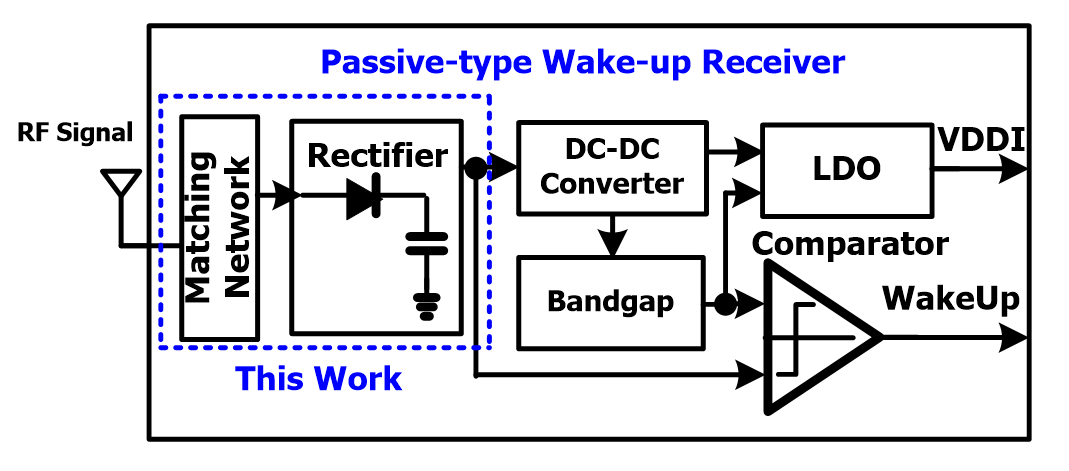## 3. Detailed Circuit Design

### 3.1 RF-to-DC Rectifier Unit Cell

Fig. 2(a) shows a voltage doubler circuit implemented using a diode as the basic structure of an RF-to-DC converter that converts RF energy into DC voltage. In the CMOS process, a diode-connected MOS transistor is used instead of a diode, as shown in Fig. 2(b). In general, the output DC voltage that can be obtained from the RF-to-DC rectifier cell in Fig. 2 is determined by the threshold voltage (Vth) of the diode or NMOS transistor used in the rectifier unit cell. Therefore, to obtain a DC voltage by rectifying the input AC signal, the amplitude of the input AC signal must be larger than the turn-on voltage of the diode or MOSFE, and the threshold voltage of the device used for the rectifier unit cell determines the output DC voltage magnitude and power conversion efficiency (PCE).

Recently, various studies have been conducted to improve the operating characteristics and PCE of the RF-to-DC rectifier unit cell, even when it has a small RF input power signal [5-8]. Although various circuit design techniques are used to improve the PCE and DC output voltage characteristics of the rectifier cell, it is difficult to overcome the limits determined by the threshold voltage of the device. The latest CMOS processes basically provide transistors with a wide variety of threshold voltages. Particularly, MOSFET devices with zero or near-zero threshold voltage are very useful for implementing a high-efficiency RF-to-DC rectifier. Therefore, to realize the proposed adaptive RF-to-DC rectifier structure to be used in a passive wake-up receiver, the rectifier unit cell is implemented using an NMOS low-threshold transistor (LVT) instead of using various Vth compensation circuit techniques, as shown in Fig. 3.

Fig. 2. Conventional rectifier unit cell as a voltage doubler.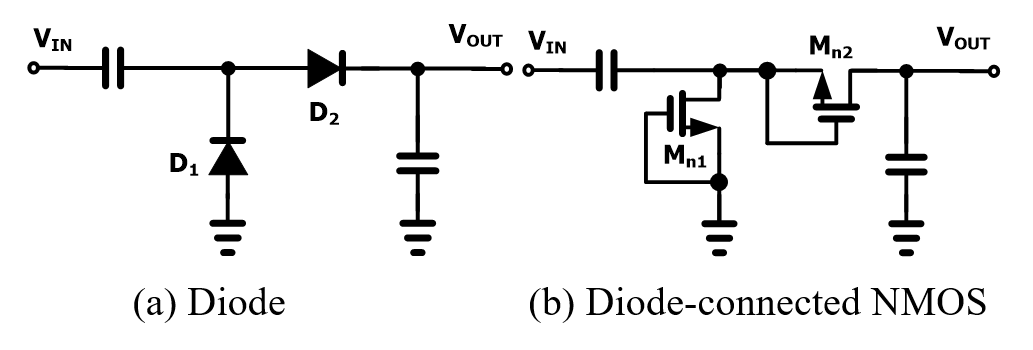Fig. 4. RF-to-DC converter equivalent circuit model including antenna and matching network.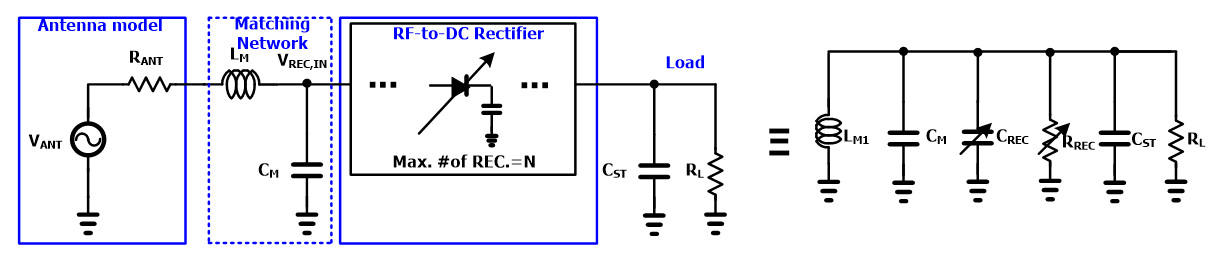Fig. 5. Simulation results as the number of rectifier unit cell increases from 1 to 4 with the fixed matching components (L$_{\mathrm{M}}$,C$_{\mathrm{M}}$).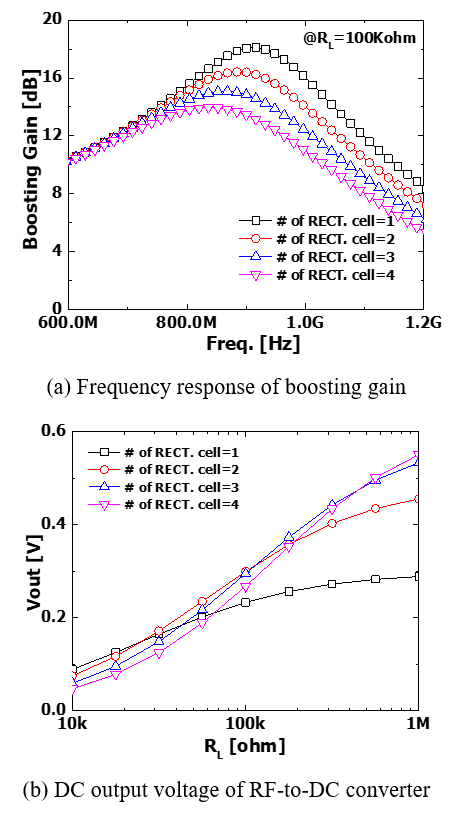### 3.2 Reconfigurable Matching Network

Typically, RF energy transmitted from the outside is transferred to the RF-to-DC rectifier through an antenna. The RF input power to the antenna is modeled as a voltage source V$_{\mathrm{ANT}}$ connected in series with a resistance R$_{\mathrm{ANT}}$ (usually 50 ${\Omega}$), as shown in Fig. 4. If the available power level delivered to the antenna is -25 dBm, the V$_{\mathrm{ANT}}$ voltage converted by Eq. (1) is very small as 35.6 mV . Thus, the NMOS transistor that constitutes the RF-to-DC rectifier may not turn on or obtain a very small DC output voltage. The wake-up receiver may not even operate.

##### (1)
$V_{ANT}=\sqrt{8\times R_{ANT}\times P_{AV}}$

For maximum RF power transmission from the antenna, an impedance matching network consisting of L$_{\mathrm{M}}$ and C$_{\mathrm{M}}$ is used. It is equivalent to a parallel RLC circuit, as shown in Fig. 4, which consists of an LC matching circuit, an equivalent capacitor (C$_{\mathrm{REC}}$), a resistor (R$_{\mathrm{REC}}$) of the RF-to-DC rectifier cell, a load resistor (R$_{\mathrm{L}}$), and load capacitor (C$_{\mathrm{ST}}$). Therefore, the magnitude of V$_{\mathrm{ANT}}$ is boosted to V$_{\mathrm{REC,IN}}$in Eq. (2) due to the loaded quality factor (Q-factor) of the parallel RLC circuit .

##### (2)
$V_{REC,IN}=\frac{V_{ANT}}{2}\sqrt{1+Q^{2}}$

As previously mentioned, when the RF input power delivered to the antenna is not large enough, the magnitude of the DC voltage that can be obtained from the rectifier unit cell is very small. Usually, even when a very small RF input signal is transferred, a multi-stage rectifier in which rectifier unit cells are connected in series is utilized to obtain the desired DC output voltage level required in an RF energy harvesting system. As the number of rectifier unit cells increases, the equivalent resistance (R$_{\mathrm{REC}}$) of the RF-to-DC converter in Fig. 4 decreases, while the capacitance (C$_{\mathrm{REC}}$) increases. Also, as the input RF frequency increases, the loaded Q-factor value of the parallel RLC equivalent circuit decreases, and the gain boosting effect by the impedance matching network decreases as well.

Fig. 5(a) shows a simulation result for the gain boosting (V$_{\mathrm{REC,IN}}$/V$_{\mathrm{ANT}}$) effect as the number of rectifier unit cells increases from 1 to 4 when the value of the optimal impedance matching network components (L$_{\mathrm{M}}$ and C$_{\mathrm{M}}$) at the RF input frequency (915 MHz) was determined. As can be seen in Fig. 5(a), the gain boosting effect by the LC matching network decreases due to the decrease in Q-factor as the rectifier unit cell increases. In addition, at the same time, as C$_{\mathrm{REC}}$ increases, the frequency response of the impedance matching network becomes lower and thus deviates from the optimum frequency characteristics. Although the increase in output DC voltage (V$_{\mathrm{out}}$) is expected due to the increase in the rectifier unit cells, it is possible to obtain a lower output DC voltage depending on the load condition.

The simulation result in Fig. 5(b) shows the output DC voltage obtained by the number of rectifier unit cells when the load resistance changes from 10 k${\Omega}$ to 1 M${\Omega}$ when the RF input power is -24 dBm. Usually, if the load resistance (R$_{\mathrm{L}}$) is large enough, the influence of the number of rectifier unit cells on the Q-factor is small, and V$_{\mathrm{out}}$ increases as the number of unit cells increases. However, as shown in Fig. 5(b), if R$_{\mathrm{L}}$ is reduced to less than 100 K${\Omega}$, the DC voltage that can be obtained with four rectifier cells may be rather small. Therefore, a method of adaptively controlling the capacitance value (C$_{\mathrm{M}}$) according to the number of used rectifier unit cells is required.

Fig. 7. V$_{\mathrm{in}}$-V$_{\mathrm{out}}$ characteristic of complementary voltage detector according to the multiplier (M) of M$_{\mathrm{n1}}$.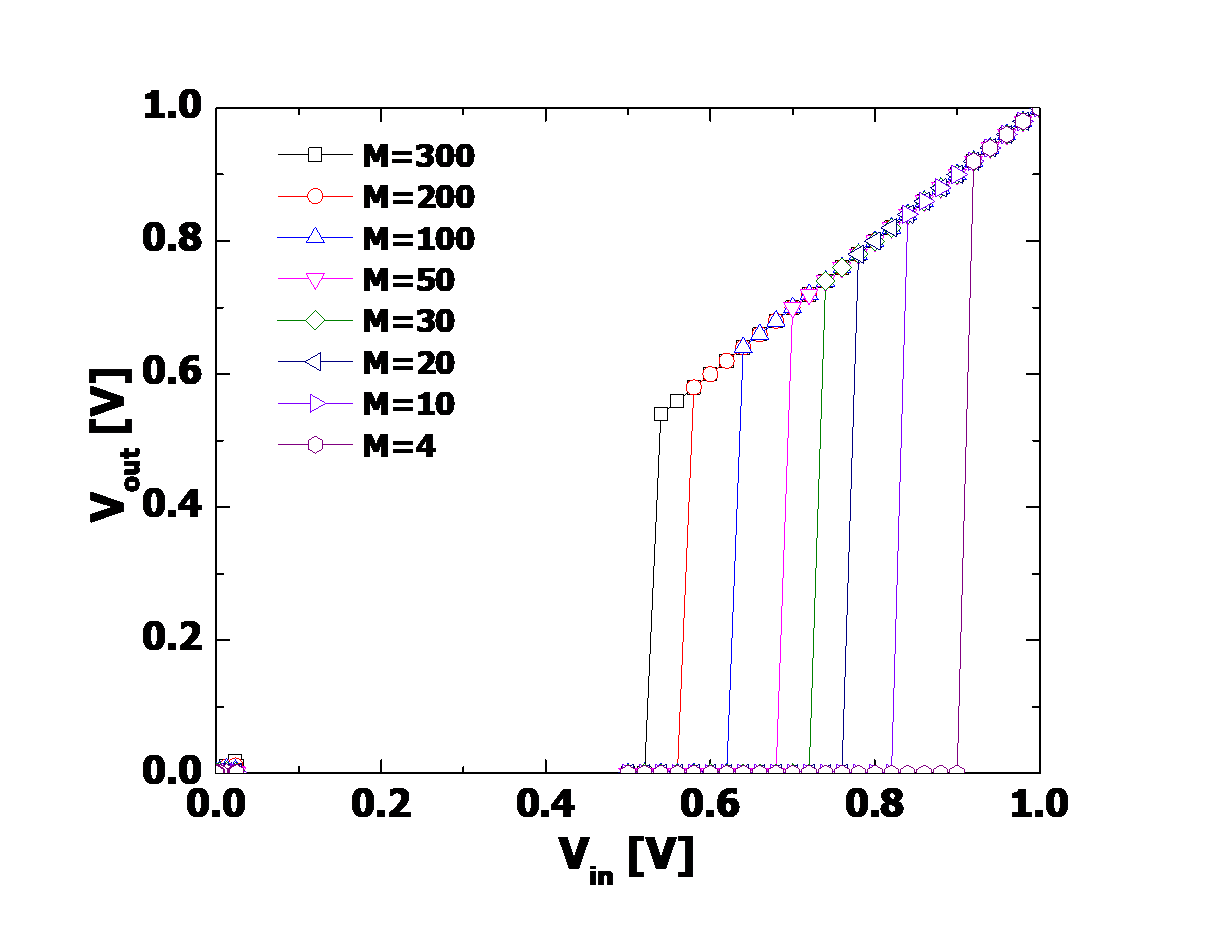Fig. 8. Block diagram of proposed RF-to-DC converter using an automatic adaptive control loop.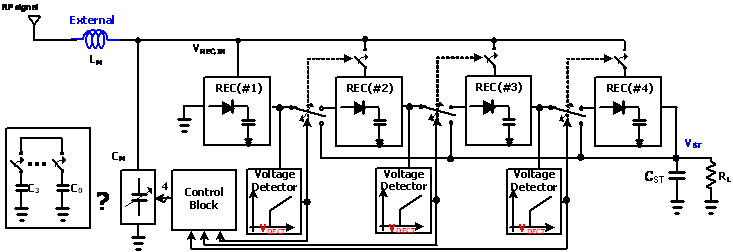### 3.3 Voltage Detector

A voltage detector is a circuit that determines whether the input voltage is higher than a reference voltage (V$_{\mathrm{REF}}$) and generally uses a comparator. However, the level detector used in a passive-type wake-up receiver must operate without an external power supply. It should also not require a reference voltage. Therefore, the voltage detector in Fig. 6 is implemented to detect the input DC voltage level without supplying external power. The level detector in Fig. 6 adopts a cross-coupled complementary structure to minimize power consumption. The detection voltage (V$_{\mathrm{DEC}}$) is determined by the size of the NMOS and PMOS, as shown in Eq. (3) .

##### (3)
$V_{DEC}=\frac{mkT}{\alpha q}ln\left(\frac{W_{n2}/L_{n2}}{W_{n1}/L_{n1}}\right)$, where $\alpha =\frac{\frac{L_{p2}}{W_{p2}}}{\frac{L_{p2}}{W_{p2}}+\frac{L_{p1}}{W_{p1}}}$

Adjusting the size ratio between the NMOS (M$_{\mathrm{n1}}$ and M$_{\mathrm{n2}}$) and PMOS (M$_{\mathrm{p1}}$ and M$_{\mathrm{P2}}$) of the voltage detector in Eq. (3) determines the desired V$_{\mathrm{DEC}}$ voltage. In addition, the V$_{\mathrm{DEC}}$ voltage can be controlled by varying the width while the length of NMOS M$_{\mathrm{n1}}$ in Fig. 6 is fixed. Fig. 7 shows the output voltage (V$_{\mathrm{out}}$) of the voltage detector according to the input voltage (V$_{\mathrm{in}}$) when the multiplier value M (the multiples of the unit transistor of the M$_{\mathrm{n1}}$ NMOS transistor) is changed from 4 to 300. The simulation result shows that as M increases, V$_{\mathrm{DEC}}$ decreases from 0.9 V to 0.5 V, as shown in Fig. 7. Therefore, it is easy to apply to various applications if the input voltage to be detected is determined by adjusting the width of the NMOS transistor M$_{\mathrm{n1}}$.

### 3.4 Proposed RF-to-DC Rectifier Structure with Adaptive Control Loop

Fig. 8 shows a block diagram of the proposed reconfigurable RF-to-DC converter structure. It consists of four rectifier unit cells, three voltage detectors, and a control block. First, the RF energy input to the antenna is converted to a DC voltage through the first rectifier unit cell, and the first voltage detector (V$_{\mathrm{D1}}$) determines whether the magnitude of this voltage is greater than or less than the desired reference DC voltage. If the RF input power is large enough, and the desired DC voltage is obtained with only the first rectifier unit cell, the voltage detector generates an output signal. The switch S$_{1}$ is directly connected to the load terminal.

Conversely, if the input RF power level is low, and there is no output signal from the voltage detector, S$_{1}$ is connected to the second rectifier unit cell, and the second voltage detector (V$_{\mathrm{D2}}$) monitors whether the desired DC voltage level is obtained. If the second voltage detector receives a lower input voltage than the desired V$_{\mathrm{REF}}$, the same operation is repeated to determine whether to connect to the final fourth rectifier unit cell. Thus, it is possible to implement an automatic reconfigurable RF-to-DC converter that determines which rectifier unit cell is used for RF-to-DC conversion using the output voltage of each rectifier unit cell.

The control logic that receives the output signals of each voltage detector generates a 4-bit control signal that adjusts the capacitor bank of the LC matching network. It also implements an adaptive control loop to compensate for changes of gain-boosting frequency response in the number of rectifier unit cells used for RF-to-DC conversion. In addition, the power conversion efficiency (PCE) typically obtained according to the number of rectifier unit cells depends on the amplitude of the RF input signal. The PCE performance of the RF-to-DC converter is more efficient when the number of rectifier cells used is one at high input RF power, and it is improved as the number of rectifier unit cell units increases with low input RF power [9,10]. Thus, by applying the proposed rectifier and adaptive control loop, high PCE characteristics can be obtained over a wide range of RF input power.

Fig. 9. Simulation results of the proposed RF-to-DC converter as the number of rectifier unit cells increases from 1 to 4.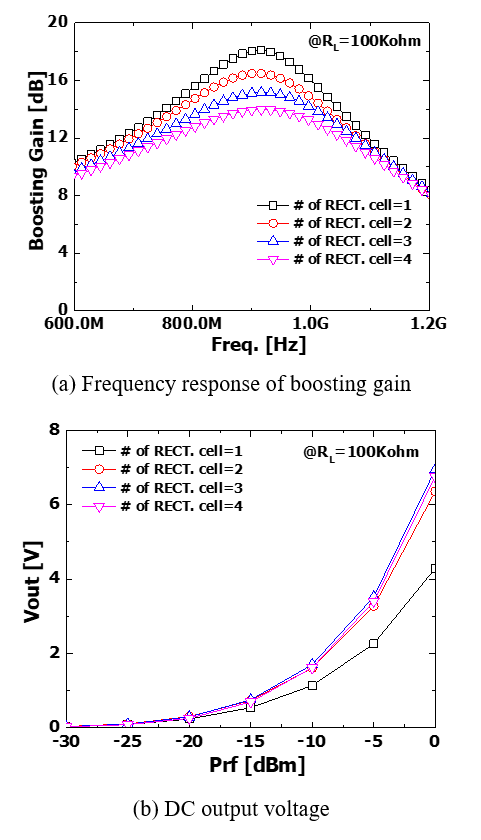Fig. 10. PCE versus input power (P$_{\mathrm{rf}}$) according to the number of rectifier unit cells from 1 to 4.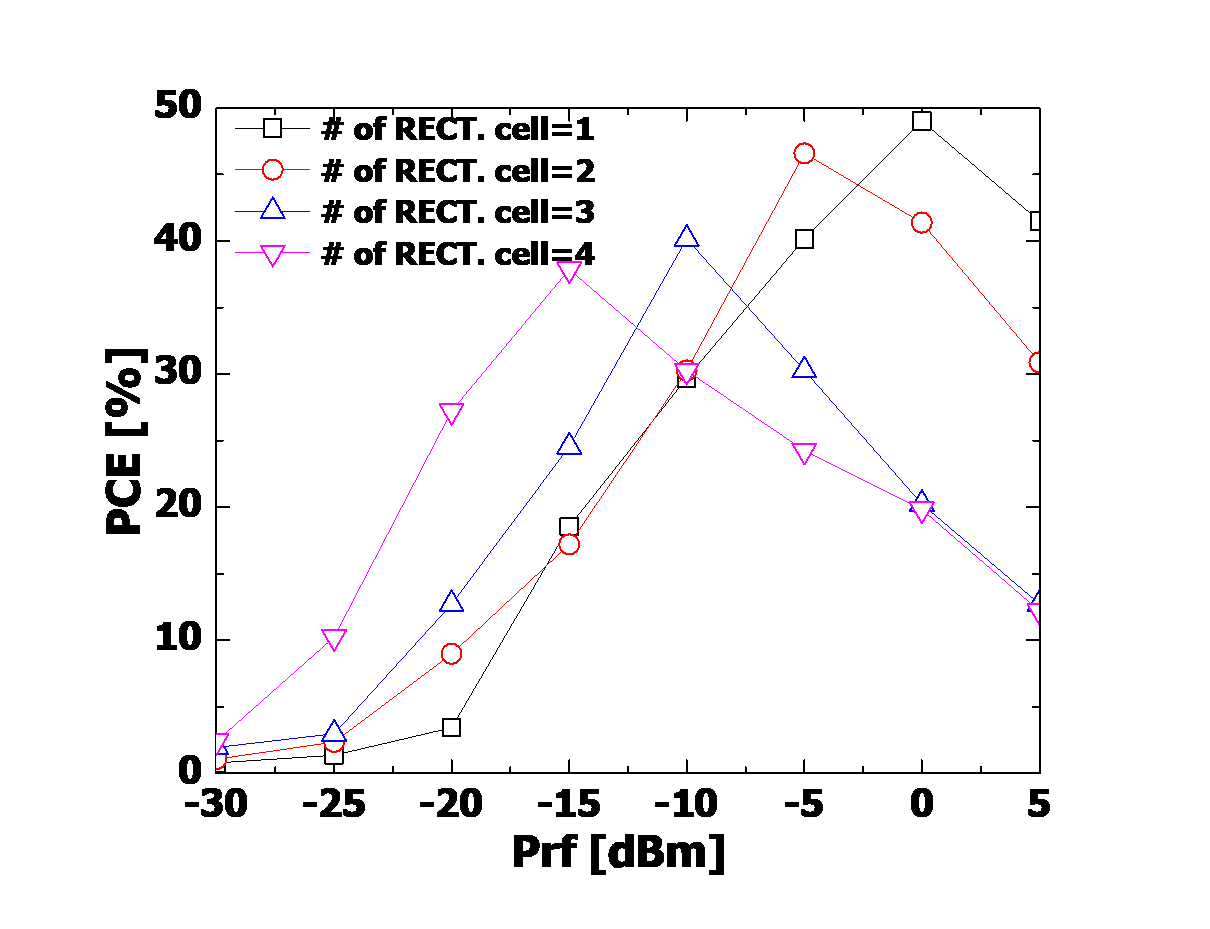## 4. Simulation Results and Discussion

The proposed structure was designed using a 0.25-${\mu}$m CMOS process, and its characteristics can be seen in Figs. 9-11. Fig. 9(a) shows the frequency response of the boosting gain of the impedance matching circuit. The response was obtained by adjusting the 4-bit capacitor bank of the LC matching network with the proposed adaptive control loop when the connection of each rectifier unit cell changes from one to four. In addition, as shown in Fig. 9(b), the characteristics of increasing output DC voltage can be obtained when the number of each rectifier unit cell increases from one to four for the RF input power, thus achieving high PCE characteristics in a wide area of RF input. Fig. 10 shows the PCE characteristics. At least 30% PCE was obtained in the range of -20 dBm to 0 dBm.

To verify the operation of control loop, the output voltage characteristics of each voltage detector were simulated at three different RF input power levels with all four rectifier unit cells connected. The reference voltage of the voltage detector was set as 0.48 V (V$_{\mathrm{DEC}}$) by fixing M of transistor M$_{\mathrm{n1}}$ in Fig. 7 at 500. At the RF input power level of -22 dBm, Fig. 11(a) shows that the output signal is not generated at voltage detectors VD$_{1}$ and VD$_{2}$, but only at VD$_{3}$. Therefore, three rectifier unit cells were configured by each voltage detector output according to the input RF power level, and the RF-to-DC converter can operate at the optimum PCE performance. Similarly, if the input signal is large enough (P$_{\mathrm{rf}}$=-16 dBm), output signals are generated from all voltage detectors, as shown in Fig. 11(c). Therefore, only the first rectifier unit cell can sufficiently obtain a desired DC voltage above the reference voltage (V$_{\mathrm{DEC}}$=0.48 V).

As shown in Fig. 11, it is demonstrated that the reconfigurable RF-to-DC converter can be implemented with the proposed adaptive control loop. Although there is a slight loss of the converted DC voltage occurs due to the addition of switches for adaptive control, the optimized PCE performance of the RF-to-DC converter can be obtained in a wide range of RF input power of about 25 dB through the operation of the automatic adaptive control loop. Therefore, the proposed rectifier structure could enable the implementation of a power-efficient passive-type wake-up receiver and various applications of WSNs consisting of IoT devices that would utilize it.

Fig. 11. Transient simulation results: the output voltages of each voltage detector through an adaptive control loop operation at three input power levels.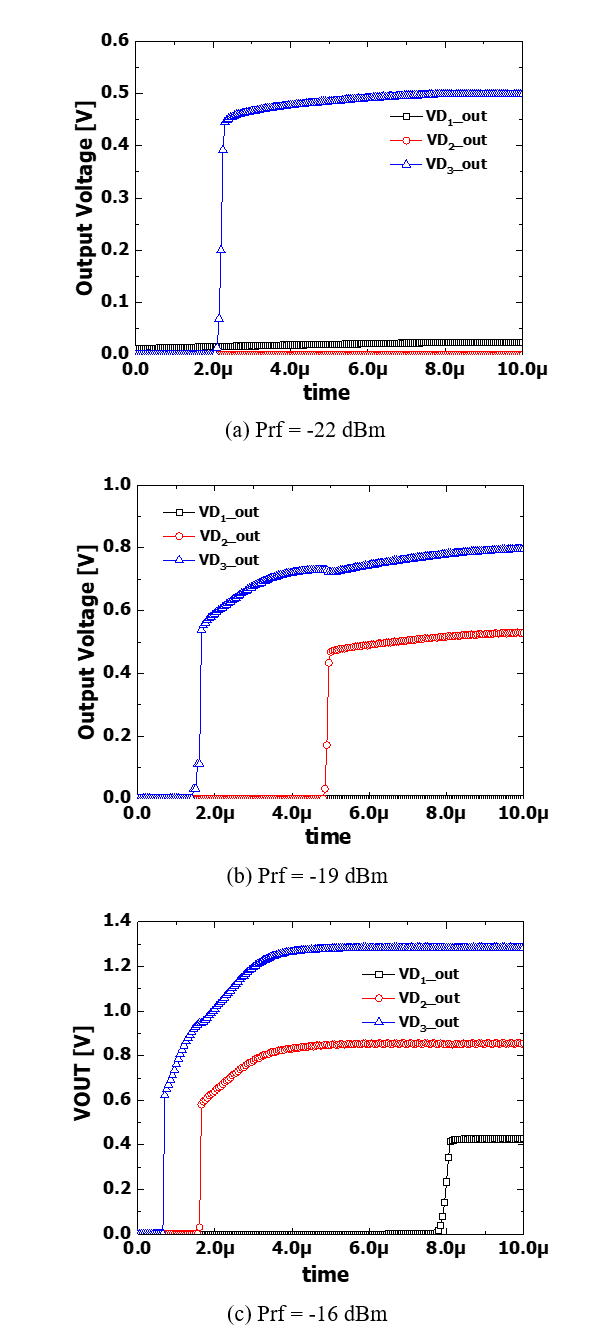## 5. Conclusion

In this paper, a reconfigurable RF-to-DC converter and automatic adaptive control loop were proposed. The converter can achieve high PCE performance in the RF input power range of more than 20dB at an RF frequency of 915 MHz. The proposed architecture was designed using 0.25-${\mu}$m CMOS technology, and its characteristics were verified through simulation. The gain boosting effect through the control of a 4-bit capacitor bank within the impedance matching network was optimized according to the number of rectifier unit cells. If the proposed reconfigurable RF-to-DC converter were used for an energy power conversion circuit of a wake-up receiver, it would be possible to operate it with a very small input signal without any external power supply. Therefore, a highly energy efficient passive-type wake-up receiver could be realized for various WSNs.

### ACKNOWLEDGMENTS

This research was supported by the Daegu University Research Grant, 2017.

### REFERENCES

1
Shekhar C., Varma S., Radhakrishna M., 2015, A 2.4 GHz passive wake-up circuit for power minimization in wireless sensor nodes, TENCON 2015 - 2015 IEEE Region 10 Conferenc,e Macao, China, pp. 1-62
Zgaren M., Sawan M., 2015, A high-sensitivity battery-less wake-up receiver for 915 MHz ISM band applications, 2015 IEEE International Conference on Electronics, Circuits, and Systems (ICECS), Cairo, Egypt, pp. 336-3393
Vamsi N., Ragulagadda S. S. R., Dutta A., Singh S. G., 2016, A -34dBm sensitivity battery-less wake-up receiver with digital decoder, 2016 IEEE Asia Pacific Conference on Circuits and Systems (APCCAS), Jeju, Korea (South), pp. 721-7244
Fumtchum A., Hutu F., Tsafack P., Villemaud G., Tanyi E., 2019, High Efficiency Rectifier for a Quasi-Passive Wakeup Radio, 2019 International Symposium on Signals, Circuits and Systems (ISSCS), Iasi, Romania, pp. 1-45
Kotani K., Sasaki A., Ito T., Nov. 2009, High-Efficiency Differential-Drive CMOS Rectifier for UHF RFIDs, in IEEE Journal of Solid-State Circuits, Vol. 44, No. 11, pp. 3011-30186
Papotto G., Carrara F., Palmisano G., Sept. 2011, A 90-nm CMOS Threshold-Compensated RF Energy Harvester, in IEEE Journal of Solid-State Circuits, Vol. 46, No. 9, pp. 1985-19977
Hameed Z., Moez K., April 2015, A 3.2 V -15 dBm Adaptive Threshold-Voltage Compensated RF Energy Harvester in 130 nm CMOS, in IEEE Transactions on Circuits and Systems I: Regular Papers, Vol. 62, No. 4, pp. 948-9568
Kim S., et al. , July 2019, A -20 to 30 dBm Input Power Range Wireless Power System With a MPPT-Based Reconfigurable 48% Efficient RF Energy Harvester and 82% Efficient A4WP Wireless Power Receiver With Open-Loop Delay Compensation, in IEEE Transactions on Power Electronics, Vol. 34, No. 7, pp. 6803-68179
Lu Y., et al. , Feb 2017, A Wide Input Range Dual-Path CMOS Rectifier for RF Energy Harvesting, in IEEE Transactions on Circuits and Systems II: Express Briefs, Vol. 64, No. 2, pp. 166-17010
Abouzied M. A., Ravichandran K., Sánchez-Sinencio E., March 2017, A Fully Integrated Reconfigurable Self-Startup RF Energy-Harvesting System With Storage Capability, in IEEE Journal of Solid-State Circuits, Vol. 52, No. 3, pp. 704-71911
Karthaus U., Fischer M., Oct. 2003, Fully integrated passive UHF RFID transponder IC with 16.7-μW minimum RF input power, in IEEE Journal of Solid-State Circuits, Vol. 38, No. 10, pp. 1602-160812
Mazzilli F., Thoppay P. E., Jöhl N., Dehollain C., 2010, Design methodology and comparison of rectifiers for UHF-band RFIDs, 2010 IEEE Radio Frequency Integrated Circuits Symposium, Anaheim, CA, USA, pp. 505-50813
Dezyani M., Ghafoorifard H., Sheikhaei S., Serdijn W. A., Oct. 2018, A 60 mV Input Voltage, Process Tolerant Start-Up System for Thermoelectric Energy Harvesting, in IEEE Transactions on Circuits and Systems I: Regular Papers, Vol. 65, No. 10, pp. 3568-3577## Author

Hyunwon Moon received a B.S. degree in radio science and engi-neering from Hanyang University, Korea, in 1997 and M.S. and Ph.D. degrees in EECS from KAIST, Korea, in 1999 and 2004, respectively. In 2004, he joined Samsung Electronics, Gyeonggi, Korea, as a senior engineer, designed multi-band multi-mode RF transceiver ICs for a cellular phone, and developed a receiver IC for a wireless connectivity system such as GPS and FM. In 2012, he joined the school of Electronic and Electric Engineering, Daegu University, Gyeongsan, Korea, as an associate professor. His research interests including CMOS RF/mmWave/analog integrated circuits and systems for wireless communications such as WSNs and 5G cellular systems.